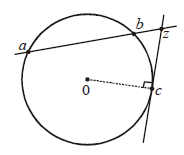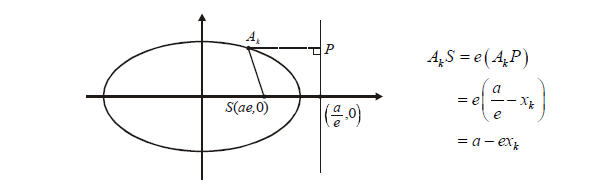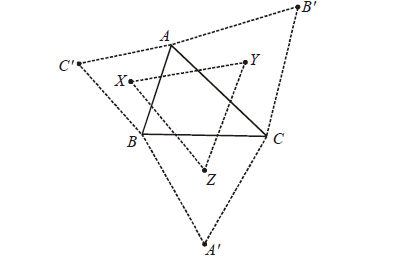# Complex Numbers Set 8

Go back to  'SOLVED EXAMPLES'

Example-  29

Three points represented by complex numbers a, b, c lie on a circle with centre origin and radius r. The tangent at C cuts the chord joining the points a, b at z. Show that \begin{align}z = \frac{{{a^{ - 1}} + {b^{ - 1}} - 2{c^{ - 1}}}}{{{a^{ - 1}}{b^{ - 1}} - {c^{ - 2}}}}\end{align}

Solution:Firstly, we note that

$|a{|^2}\; = \;|b{|^2}\; = \;|c{|^2}\; = {r^2}$

Now, z must satisfy the following two relations:

$\frac{{z - b}}{{\bar z - \bar b}} = \frac{{b - a}}{{\bar b - \bar a}}\;; \frac{{z - c}}{{\bar z - \bar c}} + \frac{c}{{\bar c}} = 0$

From the two relations, we eliminate $$\bar z$$ to obtain z:

$z = \frac{{c\left( {a\bar b - \bar ab + 2\bar c(b - a)} \right)}}{{c\left( {\bar b - \bar a} \right) + \bar c\left( {b - a} \right)}}$

Finally, we use \begin{align}\bar a = \frac{{{r^2}}}{a},\;\;\bar b = \frac{{{r^2}}}{b}\;\;{\rm{and}}\;\;\bar c = \frac{{{r^2}}}{c}\end{align}  to obtain

$z = \frac{{{a^{ - 1}} + {b^{ - 1}} - 2{c^{ - 1}}}}{{{a^{ - 1}}{b^{ - 1}} - {c^{ - 2}}}}$

Example- 30

Let $${p_k}(k = 1,\,2,......,n)$$  be the nth roots of unity. Let z = a + ib and $${A_k} = {\mathop{\rm Re}\nolimits} (z){\mathop{\rm Re}\nolimits} ({p_k}) + i\left\{ {{\mathop{\rm Im}\nolimits} (z){\mathop{\rm Im}\nolimits} ({p_k})} \right\}$$

(a)   Show that $${A_k}$$ lies on an ellipse.

Let S be the focus of the ellipse on the positive major axis.

(b)    Find $$\sum\limits_{k = 1}^n {{A_k}S}$$  (c) Find $$\sum\limits_{k = 1}^n {{{\left( {{A_k}S} \right)}^2}.}$$

Solution:   (a) Since \begin{align}{\alpha _k} = {e^{i{\theta _k}}}\;{\rm{where}}\;{\theta _k} = \frac{{2(k - 1)\pi }}{n}\end{align} , we have

${A_k} = {x_k} + i{y_k}({\rm{say}}) = \;a\cos {\theta _k} + ib\sin {\theta _k}$

Thus, \begin{align}\frac{{{x_k}}}{a} = \cos {\theta _k},\;\;\frac{{{y_k}}}{b} = \sin {\theta _k}\end{align} which implies that \begin{align}&\frac{{x_k^2}}{{{a^2}}} + \frac{{y_k^2}}{{{b^2}}} = 1\end{align}, i.e., Ak lies on an ellipse.

(b) S will be the point $$(ae,\;0)$$. However, to write the distance $${A_k}S,$$ it is easier to write it as e times the distance from the corresponding directrix:Thus, $$\sum\limits_{k = 1}^n {{A_k}S = } \sum\limits_{k = 1}^n {\left( {a - e{x_k}} \right)} = na - e\sum\limits_{k = 1}^n {{x_k}} = na$$

(c) \begin{align} \sum\limits_{k = 1}^n {{{\left( {{A_k}S} \right)}^2} } &= \sum\limits_{k = 1}^n {{{\left( {a - e{x_k}} \right)}^2} = } \;n{a^2} - 2aex\sum\limits_{k = 1}^n {{x_k} + {e^2}} \sum\limits_{k = 1}^n {x_k^2} \end{align}

\begin{align} &= n{a^2} + {a^2}{e^2}\sum\limits_{k = 1}^n {{{\cos }^2}{\theta _k}} \\&= n{a^2} + {a^2}{e^2}\;\sum\limits_{k = 1}^n {\left( {\frac{{1 + \cos 2{\theta _k}}}{2}} \right)} \\ &= n{a^2} + \frac{{n{a^2}{e^2}}}{2} + \frac{1}{2}{a^2}{e^2}\,\sum\limits_{k = 1}^n {\cos 2{\theta _k}} \end{align}

By considering the equation $${z^{n/2}} - 1 = 0$$  and its roots, we can show that $$\sum\limits_{k = 1}^n {\cos 2{\theta _k}} = 0$$, so that

$\sum\limits_{k = 1}^n {{{\left( {{A_k}S} \right)}^2}} = n{a^2} + \frac{{n{a^2}}}{2}\left( {1 - \frac{{{b^2}}}{{{a^2}}}} \right) = \frac{n}{2}\left( {3{a^2} - {b^2}} \right)$

## Example- 31

If $$\alpha$$ is a non-real complex nth root of unity, prove that:

(i)  \begin{align}&1 + 2\alpha + 3{\alpha ^2} + ... + n{\alpha ^{n - 1}} = - \frac{n}{2} - i\frac{n}{2}\cot \frac{{r\pi }}{n},r = 1,2,....,(n - 1)\end{align}

(ii)  $$1 + 3\alpha + 5{\alpha ^2} + ... + (2n - 1){\alpha ^{n - 1}} = - n - i\;n\;\cot \frac{{r\pi }}{n},r = 1,2,...,(n - 1)$$ Solution:   The given series are arithematico-geometric progressions. We can sum an AGP using the standard AGP summing technique, or using differentiation. For part - (i), we discuss both these methods:

(i)  (I) AGP summing technique

\begin{align}&\;\;\;S = 1 + 2\alpha + 3{\alpha ^2} + ... + n{\alpha ^{n - 1}}\\&\alpha S = \alpha + 2{\alpha ^2} + ... + (n - 1){\alpha ^{n - 1}} + n\,{\alpha ^n}\\ &\Rightarrow (\alpha - 1)\;S = n\,{\alpha ^n} - 1 \Rightarrow S = \frac{{n\,{\alpha ^n} - 1}}{{\alpha - 1}}\end{align}

But since $${\alpha ^n} = 1, \;\;S = \frac{n}{{\alpha - 1}}$$ . Now, all that remains is to reduce S to the standard complex form, which is straight forward. Assuming $$\alpha = {e^{\frac{{i2\pi r}}{n}}}$$ , we have

$S = - \frac{n}{2} - i\frac{n}{2}\cot \frac{{r\pi }}{n},\;\;r = 1,\;2,\;...,\;n - 1$

(II)  Differentiation

$1 + x + {x^2} + ... + {x^n} = \frac{{{x^n} - 1}}{{x - 1}}$

Differentiating this, and substituting x = a, we once again have

$1 + 2\alpha + 3{\alpha ^2} + ... + n\,{\alpha ^{n - 1}} = \frac{n}{{\alpha - 1}}$

(ii)    $$S = 1 + 3\,\alpha + 5\,{\alpha ^2} + ... + \left( {2n - 1} \right)\,{\alpha ^{n - 1}}$$

\begin{align}&\Rightarrow \left( {\alpha - 1} \right)S = \left( {2n - 1} \right){\alpha ^n} - 2\left( {\alpha + {\alpha ^2} + ... + {\alpha ^{n - 1}}} \right) - 1\\ &\qquad\qquad\;\;\quad= \left( {2n - 1} \right) - 2\left( { - 1} \right) - 1 = 2n\\ &\Rightarrow S = \frac{{2n}}{{\alpha - 1}} = - n - in\;\cot \frac{{r\pi }}{n},\;r = 1,\;2,\;...,\;n - 1\end{align}

## Example- 32

Consider a triangle ABC, on each of whose sides equilateral triangles are drawn.Prove that the centroids X, Y, Z of the three equilateral triangles themselves form an equilateral triangle.

Solution:   This very interesting result is known as the Napolean’s theorem. Although there are other ways to prove it, one of the best is through the use of complex numbers. We let the complex numbers a, b, c, $$a',\;b',\;c'$$ represent the vertices $$A,\;B,\;C,\;A',\;B',\;C'$$ respectively. Now, we make use of the fact that if $${z_1},\;{z_2},\;{z_3}$$ represent the vertices of an equilateral triangle, then $${z_1} + \omega {z_2} + {\omega ^2}\,{z_3} = 0$$ . Thus,

$a + b\omega + c'{\omega ^2} = 0 b + c\omega + a'{\omega ^2} = 0 c + a\omega + b'{\omega ^2} = 0 \ldots (1)$

Now, if complex numbers $$x,\;y,\;z$$ represent the centroids X, Y, Z respectively, then

$x = \frac{{a' + b + c}}{3},\;y = \frac{{b' + c + a}}{3},\;z = \frac{{c' + a + b}}{3} \ldots (2)$

All we need to after this is to use (1) and (2) to show that

$x + y\,\omega + z\,{\omega ^2} = 0$

This is straightforward and hence left as an exercise for the reader. The result implies that $$x,\;y,\;z$$ form the vertices of an equilateral triangle.

Learn from the best math teachers and top your exams

• Live one on one classroom and doubt clearing
• Practice worksheets in and after class for conceptual clarity
• Personalized curriculum to keep up with school

0